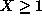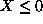Next: Search Heuristics based on Up: Constructive Search Previous: Guesses - Constraints Imposed

### MIP Search

Finite domain propagation only narrows domains, and does not guarantee to detect all inconsistencies. Thus there is no guarantee that a partial labelling (which assigns consistent values to some of the variables) can always be extended to a complete consistent labelling. However the linear constraint solver available through eplex does indeed guarantee to detect all inconsistencies between the linear constraints. On the other hand a linear solver does not take into account the constraint that certain variables can only take integer values, thus it can return proposed solutions in which non-integer values are proposed for integer variables. The linear solver can efficiently find an optimal solution to the problem in which integrality constraints on the variables are ignored. Such an optimum is termed an optimum of the ``continuous relaxation'' of the problem, or just the ``relaxed optimum'' for short.

This suggests a different search mechanism, in which a new constraint is added to exclude the non-integer value in the relaxed optimum returned by the linear constraint solver. If the value for integer variable X was 0.5 in the relaxed optimum, for example, a new constraintmight be added. Since this excludes other feasible solutions such as X=0, this new constraint is only a guess, and if it turns out to be a bad guess then the alternative constraintis posted instead.

This is the search method used in MIP when optimize is called in the eplex library.

Joachim Schimpf
Wed Sep 3 18:07:19 BST 1997